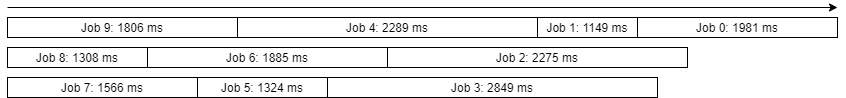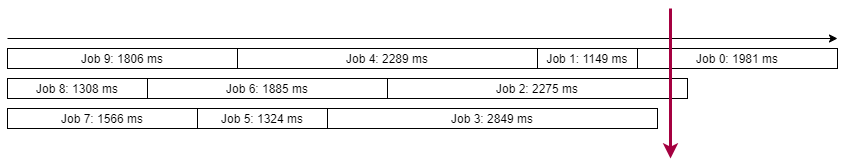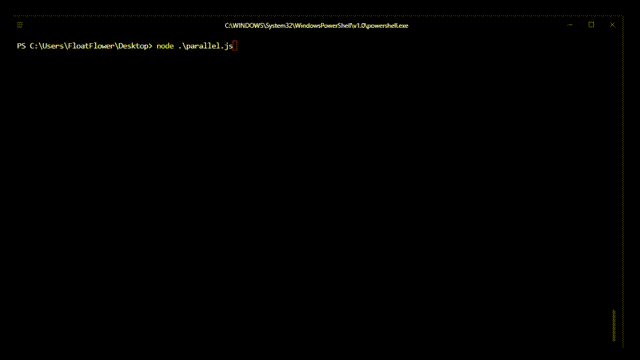In this article, I am going to show you how to use Node.js to implement it which I mentioned above.

### Static number of jobs

In the first part, I will show you the “Parallelization with a static number of jobs”, which means the number of jobs is pre-defined, and the new jobs will never be pushed to the job queue until the program is terminated.

First of all, we are going to define the job we are going to run, to avoid too complicated code to understand, I will use `Promise` and `setTimeout` to simulate the asynchronous job:

``````const buildJob = (jobId, executionTime) => {
return () => {
console.log(`[Job \${jobId}] Start.`)
return new Promise(resolve => {
setTimeout(() => {
console.log(`[Job \${jobId}] End, it takes \${executionTime} ms.`)
resolve();
}, executionTime);
})
}
}

// we expect that each job should output the message like this:
// [Job 1] Start.
// [Job 1] End, it takes 2512 ms.
``````

In the five lines of code above, the `buildJob()` function will return a function that returns a `Promise` after calling it. and then we can use this function to build a series of jobs.

``````const randomExecutionTimes = (new Array(10))
.fill()
.map(() => Math.floor(Math.random() * 2000 + 1000))
const jobs = randomExecutionTimes
.map((executionTime, index) => buildJob(index, executionTime))
``````

Now, we have got 10 jobs which have totally random execution time from 1000ms to 3000ms, let’s make them run parallelly.

``````const jobsQueue = jobs.map(job => job);
``````

First of all, we need to build a new array named `jobsQueue` because we must keep the `jobs` unchanged, and we see the start of this newly created array as tail, and the end of the array as head, so only we need to do is `pop()` the last element of the array and call the job functions.

Now, we gonna write a function call `startJob()` that starts a job at the head of the queue.

``````function startJob() {
const job = jobsQueue.pop();
// start another job after finishing the previous one.
if(job) return job.then(startJob)
}
``````

Then, let’s start running the job.

``````// We use the variable to define the max number of jobs
const MAX_PARALLEL_NUMBER = 3;
// Then use the for loop to start
for(let i = 0; i < MAX_PARALLEL_NUMBER; i ++) {
startJob();
}
``````

Finally, let’s combine all parts together.

``````const buildJob = (jobId, executionTime) => {
return () => {
console.log(`[Job \${jobId}] Start.`)
return new Promise(resolve => {
setTimeout(() => {
console.log(`[Job \${jobId}] End, it takes \${executionTime} ms.`)
resolve();
}, executionTime);
})
}
}

const randomExecutionTimes = (new Array(10))
.fill()
.map(() => Math.floor(Math.random() * 2000 + 1000))
const jobs = randomExecutionTimes
.map((executionTime, index) => buildJob(index, executionTime))

const jobsQueue = jobs.map(job => job);

function startJob() {
const job = jobsQueue.pop();
if(job) return job.then(startJob)
}

const MAX_PARALLEL_NUMBER = 3;
for(let i = 0; i < MAX_PARALLEL_NUMBER; i ++) {
startJob();
}
``````

Ok, done. Let’s run the program.

``````[Job 9] Start.
[Job 8] Start.
[Job 7] Start.
[Job 8] End, it takes 1308 ms.
[Job 6] Start.
[Job 7] End, it takes 1566 ms.
[Job 5] Start.
[Job 9] End, it takes 1806 ms.
[Job 4] Start.
[Job 5] End, it takes 1324 ms.
[Job 3] Start.
[Job 6] End, it takes 1885 ms.
[Job 2] Start.
[Job 4] End, it takes 2289 ms.
[Job 1] Start.
[Job 2] End, it takes 2275 ms.
[Job 0] Start.
[Job 1] End, it takes 1449 ms.
[Job 3] End, it takes 2849 ms.
[Job 0] End, it takes 1981 ms.
``````

By the way, let’s make a little change to see how long it takes to finish this program.

``````const MAX_PARALLEL_NUMBER = 3;
// save the time when starting
const startTime = Date.now();
const executors = [];
for(let i = 0; i < MAX_PARALLEL_NUMBER; i ++) {
executors.push(startJob());
}
// wait for all jobs be done.
Promise.all(executors).then(() => {
const endTime = Date.now();
console.log(`All jobs take \${endTime - startTime} ms running.`)
})
``````

And this is the output of this change:

``````[Job 9] Start.
[Job 8] Start.
[Job 7] Start.
[Job 8] End, it takes 1308 ms.
[Job 6] Start.
[Job 7] End, it takes 1566 ms.
[Job 5] Start.
[Job 9] End, it takes 1806 ms.
[Job 4] Start.
[Job 5] End, it takes 1324 ms.
[Job 3] Start.
[Job 6] End, it takes 1885 ms.
[Job 2] Start.
[Job 4] End, it takes 2289 ms.
[Job 1] Start.
[Job 2] End, it takes 2275 ms.
[Job 0] Start.
[Job 1] End, it takes 1449 ms.
[Job 3] End, it takes 2849 ms.
[Job 0] End, it takes 1981 ms.
All jobs take 7476 ms running.
``````

Pretty cool, we make the jobs run parallelly. I make a chart to show how the jobs are done, when there are only three jobs run simultaneously. This graph shows that all jobs can be done at 7225ms in theory, which almost equals to the result: 7476ms.### Dynamic number of jobs

The first part is just an example, it shares the concept of parallelizing jobs, however, in some production scenery, we face to more complex conditional than this, isn’t it? In the second part, we are going to move further, I’ll show you the “Parallelization with dynamic number of jobs”, which means the jobs will be pushed to queue during the program runs.

We can not ensure when jobs will be pushed to the queue is the main difference between dynamic queue and static one. In the previous section, we start the next job from the previous job after finishing execution. However, in the dynamic queue, the jobs could be pushed when there are only 1 or 2 jobs are still running.The graph shows that if we only use the method provided in the first section. When the jobs are pushed to the queue at the timing which is marked in the graph, it has only two parallel jobs left, because after Job3 is done, `startJob()` will be called afterward but get nothing to run.

Let’s make some changes from the code written in the first section. First, we gonna implement a function called `addJob()`.

``````function addJob(job) {
jobsQueue.unshift(job);
return startJob();
}
``````

This function is very simple, the most important part is calling `startJob()` after adding a job to the queue. The purpose that calling `startJob()` afterward is to make sure that every job has its `startJob()`. To put it differently, if there is any job in the queue, then `startJob()` call by the previous job, otherwise, the `startJob()` is called after adding a job to the queue.

Now, we are going to modify the `startJob()` function, because `startJob()` will to run the job after being called.

``````let concurrentJobsCount = 0;
function startJob() {
if(concurrentJobsCount >= MAX_PARALLEL_NUMBER) {
const job = jobsQueue.pop();
if(job) {
concurrentJobsCount ++;
return job.then(startJob).finally(() => {concurrentJobsCount --})
}
}
}
``````

We have almost done. Because this is a single-process and single-thread application, so we don’t need to see `concurrenctJobsCount` as a critical section variable, so just use it directly to count how many jobs are running.

In the end, left write a simple script to add jobs to the queue when running.

``````// because we have 10 jobs already, so jobId start from 11.
let jobId = 11;

// this function will create a job and append to the queue every 2000ms.
function createRuntimeJob() {
setTimeout(() => {
const job = buildJob(jobId ++, Math.floor(Math.random() * 2000 + 1000));
createRuntimeJob();
}, 2000)
}

createRuntimeJob();
``````

Let’s combine the code again:

``````const buildJob = (jobId, executionTime) => {
return () => {
console.log(`[Job \${jobId}] Start.`)
return new Promise(resolve => {
setTimeout(() => {
console.log(`[Job \${jobId}] End, it takes \${executionTime} ms.`)
resolve();
}, executionTime);
})
}
}

const randomExecutionTimes = (new Array(10))
.fill()
.map(() => Math.floor(Math.random() * 2000 + 1000))
const jobs = randomExecutionTimes
.map((executionTime, index) => buildJob(index, executionTime))

const jobsQueue = jobs.map(job => job);

const MAX_PARALLEL_NUMBER = 3;
let concurrentJobsCount = 0;
function startJob() {
if(concurrentJobsCount < MAX_PARALLEL_NUMBER) {
const job = jobsQueue.pop();
if(job) {
concurrentJobsCount ++;
return job().then(startJob).finally(() => {concurrentJobsCount --})
}
}
}

for(let i = 0; i < MAX_PARALLEL_NUMBER; i ++) {
startJob();
}

jobsQueue.unshift(job);
return startJob();
}

let jobId = 11;
function createRuntimeJob() {
setTimeout(() => {
const job = buildJob(jobId ++, Math.floor(Math.random() * 2000 + 1000));
createRuntimeJob();
}, 2000)
}

createRuntimeJob();
``````We make all jobs run parallelly and it looks good! After deleting the code that do job generation, we have few lines of code left:

``````// jobs varaiable is defined by yourself.
const jobsQueue = jobs.map(job => job);

let concurrentJobsCount = 0;
function startJob() {
if(concurrentJobsCount < MAX_PARALLEL_NUMBER) {
const job = jobsQueue.pop();
if(job) {
concurrentJobsCount ++;
return job().then(startJob).finally(() => {concurrentJobsCount --})
}
}
}

const MAX_PARALLEL_NUMBER = 3;
for(let i = 0; i < MAX_PARALLEL_NUMBER; i ++) {
startJob();
}